#### 期刊菜单

Fast Load Transient Response LDO with Slew Rate Enhancement Circuit
DOI: 10.12677/OJCS.2020.92003, PDF, HTML, XML, 下载: 457  浏览: 1,264

Abstract: Because of the rapid development of SoC (System on a Chip), the requirements to LDO (Low Dropout Linear Regulator) chip become more and more rigorous. Lower power consumption, fewer periph-eral devices, faster response speed, higher conversion efficiency and power supply rejection ratio have become hotspot of LDO chip. In this paper, a large capacitance free LDO is proposed, which us-es slew rate enhancement technology and dynamic bias techniques to optimize slew rate of the LDO, so the transient response of the output voltage becomes fast enough when the load current changes.

1. 引言

2. 电路架构及工作原理

LDO电路架构如图1所示。其中电阻R1~R4及功率管M0、电容C1、误差放大器EA组成主环路，SRE模块为摆率增强电路模块，输出电压发生变化时，该模块会输出一股电流对功率管的栅极进行充电或者放电操作，加快功率管栅极电压的变化，从而提高LDO的负载瞬态响应能力 。另外该SRE电路具有很好的移植性，可以应用于所有其他LDO电路之中。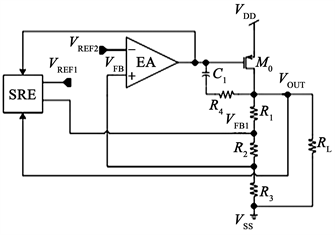Figure 1. Fast load transient response without external large capacitance LDO architecture with swing rate enhancement circuit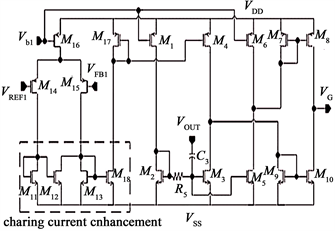Figure 2. Specific circuit diagram of swing rate enhancement circuit

3. 要点分析和具体实现Figure 3. Relation between angular frequency and loop bandwidth of high pass filter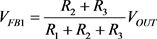(1)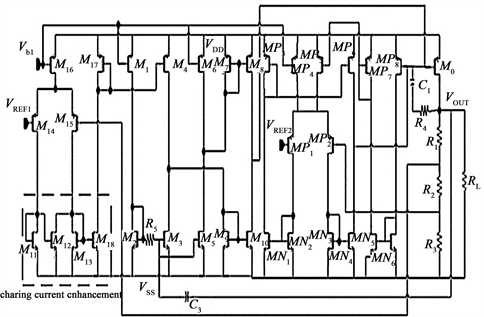Figure 4. Overall circuit diagram of off-chip capacitive LDO for fast load transient response

4. 稳定性分析

LDO由两级放大器构成环路，其中第一级为MP1~MP6及MN1~MN4构成的误差放大器EA，第二级为由功率管及输出电阻构成的功率管级，他们连同由R1~R3组成的反馈网络构成LDO的主环路。为了实现该LDO的稳定性，该LDO采用密勒补偿的方法，将主极点设置在功率管的栅极，并且采用调零电阻对环路相位裕度进行优化，从而实现环路高带宽。该环路的小信号等效电路如图5所示。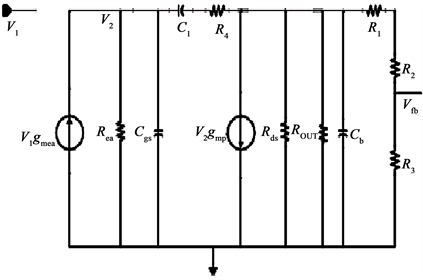Figure 5. Small external capacitance LDO signal equivalent circuit with fast load transient response

$L\left(s\right)=\frac{{v}_{fb}}{{v}_{1}}=\frac{{g}_{mea}{R}_{ea}{g}_{mp}{R}_{O_ALL}F\left(1-\left(1/{g}_{mp}-{R}_{4}\right){C}_{1}S\right)}{\left[{g}_{mp}{R}_{O_ALL}{C}_{1}{R}_{ea}S+1\right]\left(1+\frac{{C}_{1}}{{g}_{mp}}S\right)}$ (2)$F=\frac{{R}_{3}}{{R}_{1}+{R}_{2}+{R}_{3}}$ (3)

${p}_{1}=-\frac{1}{{g}_{mp}{R}_{O_ALL}{C}_{1}{R}_{ea}}$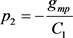(4)

${z}_{1}=-\frac{1}{\left({R}_{4}-1/{g}_{mp}\right){C}_{1}}$ (5)

5. 仿真与分析

5.1. 直流特性仿真与分析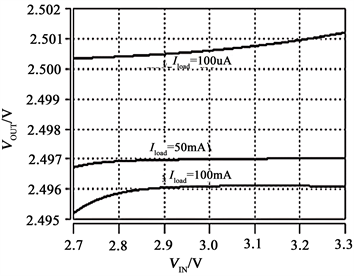Figure 6. Simulation wave map of linear adjustment rate of fast load transient response LDO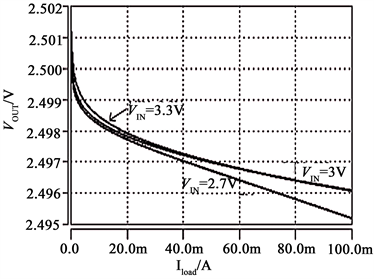5.2. 交流特性仿真与分析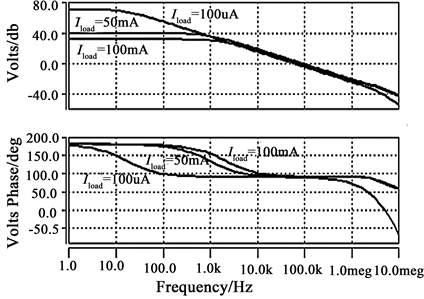Figure 8. Spectrum frequency and phase frequency characteristic curves of fast load transient response LDO under different load conditions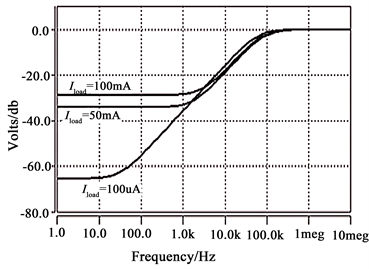Figure 9. PSRR simulation curve of fast load transient response LDO under different loads

5.3. 瞬态特性仿真与分析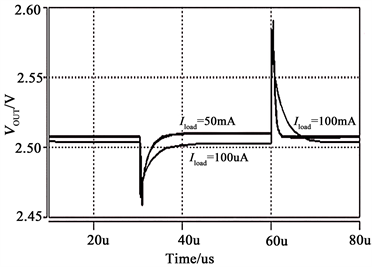Figure 10. Linear transient simulation of rapid-load transient response to LDO under different loads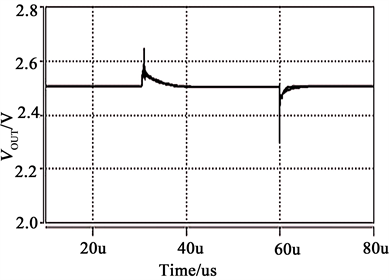Figure 11. Load transient simulation curve of fast load transient response to LDO under different power supply voltage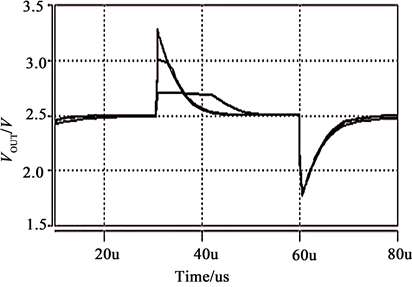Figure 12. Load transient simulation curve of different power supply voltage-free rate enhancement circuits and dynamic bias circuits LDO

6. 结论

  葛瑞. 低过冲电压LDO线性稳压器的设计和研究[D]: [硕士学位论文]. 成都: 电子科技大学, 2012: 1-5.  范东风. 高性能LDO线性稳压器的设计[D]: [硕士学位论文]. 大连: 大连理工大学, 2008: 1-3.  马勋. LDO线性降压变换器的研究[D]: [硕士学位论文]. 成都: 电子科技大学,2004: 1-5.  王忆. 高性能低压差线性稳压器研究与设计[D]: [博士学位论文]. 杭州: 浙江大学, 2010: 1-2.  Lau, S.K., Mok, P.K.T. and Leung, K.N. (2007) A Low-Dropout Regulator for SoC with Q-Reduction. IEEE Journal of Solid-State Circuits, 42, 658-664. https://doi.org/10.1109/jssc.2006.891496  Man, T.Y., Leung, K.N., Leung, C.Y., et al. (2008) Development of Single-Transistor-Control LDO Based on Flipped Voltage Follower for SoC. IEEE Transactions on Circuits and Sys-tems I: Regular Papers, 55, 1392-1401. https://doi.org/10.1109/tcsi.2008.916568  Hazucha, P., Karnik, T., Bloechel, B.A., et al. (2005) Area-Efficient Linear Regulator with Ultra-Fast Load Regulation. IEEE Journal of Solid-State Circuits, 40, 933-940. https://doi.org/10.1109/jssc.2004.842831